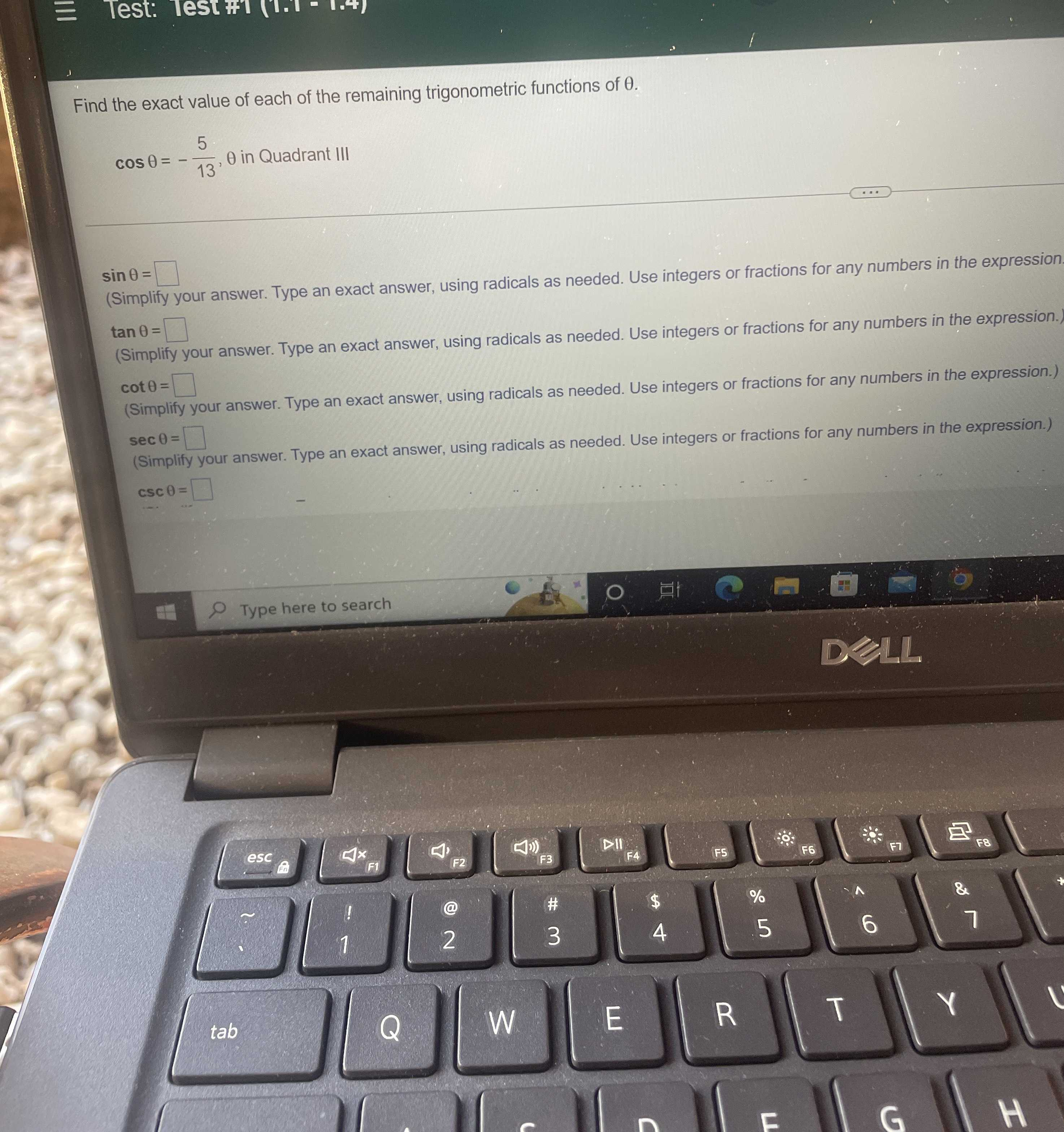### Still have math questions?

Trigonometry
QuestionFind the exact value of each of the remaining trigonometric functions of $$\theta$$ .

$$\cos \theta = - \frac { 5 } { 13 } , \theta$$ in Quadrant III

$$\sin \theta = \square$$

$$\tan \theta = \square$$

$$\cot \theta = \square$$

$$\sec \theta = \square$$

$$\csc \theta = \square$$

sin$$\theta$$=$$\frac { 12} { 13}$$
tan$$\theta$$=$$- \frac { 12} { 5}$$
cot$$\theta$$=$$- \frac { 5} { 12}$$
sec$$\theta$$=$$- \frac { 13} { 5}$$
csc$$\theta$$=$$\frac { 13} { 12}$$